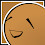# JavaScript async/await 的奇淫技巧

## 實現 delay 函數

``````// 實現一個等待函數
const delay = (interval) => {
return new Promise((resolve) => {
setTimeout(resolve, interval);
});
};

const main = async () => {
console.log('Starting...');

// 等待五秒
await delay(5000);

console.log('Done after five seconds')
};

main();``````

## 與 .map() 的組合技

JavaScript 陣列裡常使用的 `.map()` 方法，但是 `.map()` 方法內的處理函數是同步的（synchronous），也就是如果我們想在裡面跑非同步的邏輯，是沒辦法等到我們非同步的工作完成的。

``````const arr = [ 1, 2, 3, 4, 5 ];

let results = arr.map((num) => {
return num + 1;
});

// [ 2, 3, 4, 5, 6 ]
console.log(results);``````

``````const asyncWorker = async (num) => {
// 非同步的工作，會做一段時間
};

let results = arr.map(async (num) => {
// 等待非同步工作完成
await asyncWorker(num);

return num + 1;
});``````

## 等 .map() 裡的所有工作處理完

``````const asyncWorker = async (num) => {
// 非同步的工作，會做一段時間
};

let jobs = arr.map(async (num) => {
// 等待非同步工作完成
await asyncWorker(num);

return num + 1;
});

// 當所有工作完成後，顯示執行內容
Promise.all(jobs).then((results) => {
// [ 2, 3, 4, 5, 6 ]
console.log(results);
});``````

## 用 await 取代 promise.then() 的使用方式

``````Promise.all(arr.map(async (num) => {
// 等待非同步工作完成
await asyncWorker(num);

return num + 1;
})).then((results) => {
// [ 2, 3, 4, 5, 6 ]
console.log(results);
});``````

``````const main = async () => {

// 改用 await 等待 Promise 內的工作全部完成
let results = await Promise.all(arr.map(async (num) => {
// 等待非同步工作完成
await asyncWorker(num);

return num + 1;
}));

// [ 2, 3, 4, 5, 6 ]
console.log(results);
};

main();``````

## 與 .reduce() 的組合技

``````const arr = [ 1, 2, 3, 4, 5 ];

// 將陣列所有數值一一加總
let result = arr.reduce((accumulation, num) => {
return accumulation + num;
}, 0);

// 15
console.log(result);``````

``````const arr = [ 1, 2, 3, 4, 5 ];

const main = async () => {

// 將陣列所有數值一一加總
let result = await arr.reduce(async (prev, num) => {

// 等待前一個工作完成，並得到前個工作的結果
let accumulation = await prev;

return accumulation + num;
}, Promise.resolve(0));

// 15
console.log(result);
};

main();``````

``````const arr = [ 1, 2, 3, 4, 5 ];

const main = async () => {

// 將陣列所有數值一一加總
let result = await arr.reduce(async (prev, num) => {

// 等待前一個工作完成，並得到前個工作的結果
let accumulation = await prev;

// 非同步工作：等一秒
await delay(1000);

return accumulation + num;
}, Promise.resolve(0));

// 15
console.log(result);
};

main();``````

## 後記

### 留言

1.這篇超級實用，感謝彙整

2.很實用 ，謝謝大大的分享 幫助我很多